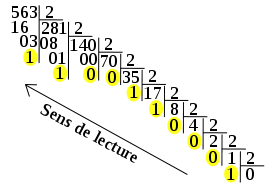### R convert IPv4 adresses to binary

###### Mon 07 July 2014

[caption id="" align="alignright" width="270"]binary conversion (Photo credit: Wikipedia)[/caption]

I want to retrieve the binary representation (as a string of 0 and 1) of an IPv4 address (human readable string representation) using only R functions.

The following steps and functions can be used:

step tool
separate each octet strsplit(x, split="[.]")
convert integer to binary as.integer(intToBits(x))
reunite all the octet paste0(x, collapse="")

the result is:

```##
# give the representation of an IPv4 in binary
# @param ip ipv4
# @return a string made of 1 and 0
manu.IPv4tobin <- function(ip){
r=unlist(strsplit(ip, split="[.]"))
paste0(unlist(lapply(r, function(x){rev(as.integer(intToBits(x)))[25:32]})), collapse="")
}
```

Category: how to Tagged: IP address IPv4 programming R